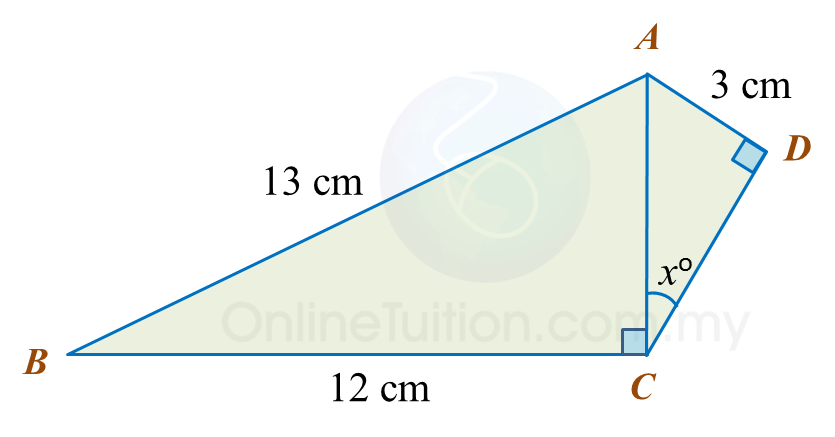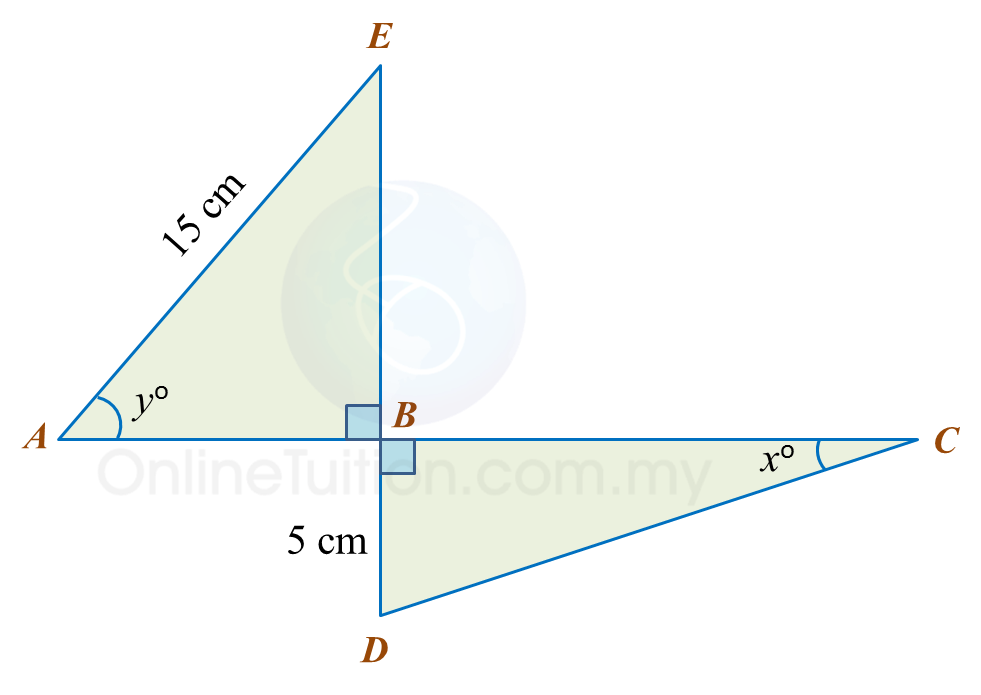15.2.1 Trigonometry, PT3 Focus Practice

15.2.1 Trigonometry, PT3 Focus Practice

Question 1:
Diagram below shows a right-angled triangle ABC.It is given that  $\mathrm{cos}{x}^{o}=\frac{5}{13}$ , calculate the length, in cm, of AB.

Solution:

Question 2:
In the diagram, PQR and QTS are straight lines.It is given that $\mathrm{tan}y=\frac{3}{4}$ , calculate the length, in cm, of RS.

Solution:

Question 3:
In the diagram, PQR is a straight line.It is given that   $\mathrm{cos}{x}^{o}=\frac{3}{5}$ , hence sin yo =

Solution:

Question 4:
Diagram below consists of two right-angled triangles.Determine the value of cos xo.

Solution:

Question 5:
Diagram below consists of two right-angled triangles ABE and DBC.
ABC and EBD are straight lines.It is given that
(a) Find the value of tan xo.
(b)   Calculate the length, in cm, of ABC.

Solution:
(a)

(b)